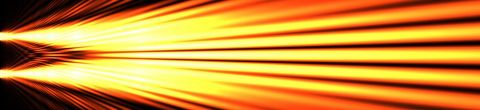# Quantum MechanicsWinter, 2012

Quantum theory governs the universe at its most basic level. In the first half of the 20th century physics was turned on its head by the radical discoveries of Max Planck, Albert Einstein, Niels Bohr, Werner Heisenberg, and Erwin Schroedinger. An entire new logical and mathematical foundation—quantum mechanics—eventually replaced classical physics. We will explore the quantum world, including the particle theory of light, the Heisenberg Uncertainty Principle, and the Schrödinger Equation.

## Lectures in this Course

1. ### Introduction to quantum mechanics

Professor Susskind opens the course by describing the non-intuitive nature of quantum mechanics.  With the discovery of quantum mechanics, the fundamental laws of physics moved into a realm that defies human intuition or visualization.  Quantum... [more]
2. ### The basic logic of quantum mechanics

Professor Susskind introduces the simplest possible quantum mechanical system: a single particle with spin.  He presents the fundamental logic of quantum mechanics in terms of preparing and measuring the direction of the spin.  This fundamental... [more]
3. ### Vector spaces and operators

Professor Susskind elaborates on the abstract mathematics of vector spaces by introducing the concepts of basis vectors, linear combinations of vector states, and matrix algebra as it applies to vector spaces.  He then introduces linear operators... [more]
4. ### Time evolution of a quantum system

Professor Susskind opens the lecture by presenting the four fundamental principles of quantum mechanics that he touched on briefly in the last lecture.  He then discusses the evolution in time of a quantum system, and describes how the classical... [more]
5. ### Uncertainty, unitary evolution, and the Schrödinger equation

Professor Susskind begins the lecture by introducing the Heisenberg uncertainty principle and explains how it relates to commutators. He proves that two simultaneously measurable operators must commute. If they don't then the observables... [more]
6. ### Entanglement

Professor Susskind begins the lecture with a review of the problem of a single spin in a magnetic field. He re-emphasizes that observables corresponding to the Pauli sigma matrices do not commute, which implies that they obey the uncertainty... [more]
7. ### Entanglement and the nature of reality

This lecture takes a deeper look at entanglement.  Professor Susskind begins by discussing the wave function, which is the inner product of the system's state vector with the set of basis vectors, and how it contains probability amplitudes for the... [more]
8. ### Particles moving in one dimension and their operators

Professor Susskind opens the lecture by examining entanglement and density matrices in more detail.  He shows that no action on one part of an entangled system can affect the statistics of the other part.  This is the principle of locality and is... [more]
9. ### Fourier analysis applied to quantum mechanics and the uncertainty principle

Professor Susskind opens the lecture with a review of the entangled singlet and triplet states and how they decay.  He then shows how Fourier analysis can be used to decompose a typical quantum mechanical wave function.
10. ### The uncertainty principle and classical analogs

Professor Susskind begins the final lecture of the course by deriving the uncertainty principle from the triangle inequity.  He then shows the correspondence between the motion of wave packets and the classical equations of motion.  The expectation... [more]